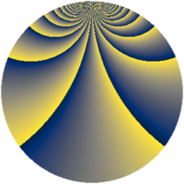# Properties

 Label 2001.2.nLevel $2001$ Weight $2$ Character orbit 2001.n Rep. character $\chi_{2001}(262,\cdot)$ Character field $\Q(\zeta_{11})$ Dimension $1120$ Sturm bound $480$

# Related objects

## Defining parameters

 Level: $$N$$ $$=$$ $$2001 = 3 \cdot 23 \cdot 29$$ Weight: $$k$$ $$=$$ $$2$$ Character orbit: $$[\chi]$$ $$=$$ 2001.n (of order $$11$$ and degree $$10$$) Character conductor: $$\operatorname{cond}(\chi)$$ $$=$$ $$23$$ Character field: $$\Q(\zeta_{11})$$ Sturm bound: $$480$$

## Dimensions

The following table gives the dimensions of various subspaces of $$M_{2}(2001, [\chi])$$.

Total New Old
Modular forms 2440 1120 1320
Cusp forms 2360 1120 1240
Eisenstein series 80 0 80

## Trace form

 $$1120q - 104q^{4} + 8q^{5} + 8q^{6} + 8q^{7} - 112q^{9} + O(q^{10})$$ $$1120q - 104q^{4} + 8q^{5} + 8q^{6} + 8q^{7} - 112q^{9} + 16q^{10} + 32q^{11} + 8q^{13} + 16q^{14} + 8q^{15} - 88q^{16} - 28q^{17} + 48q^{19} - 104q^{20} + 8q^{21} + 56q^{22} + 20q^{23} + 24q^{24} - 88q^{25} + 56q^{26} - 144q^{28} + 16q^{30} - 20q^{31} + 40q^{32} - 28q^{34} - 32q^{35} - 104q^{36} - 64q^{37} + 16q^{38} + 84q^{40} - 16q^{41} - 180q^{42} + 24q^{43} + 112q^{44} + 8q^{45} + 52q^{46} - 120q^{47} - 40q^{49} + 56q^{50} + 32q^{51} - 148q^{52} - 64q^{53} - 36q^{54} + 72q^{55} + 48q^{56} - 72q^{57} - 24q^{59} + 20q^{60} + 8q^{61} + 168q^{62} + 8q^{63} - 184q^{64} + 112q^{65} + 16q^{66} + 40q^{67} + 112q^{68} + 24q^{69} - 24q^{70} + 32q^{71} + 40q^{73} + 64q^{74} + 32q^{75} - 220q^{76} + 136q^{77} + 16q^{78} - 144q^{79} + 200q^{80} - 112q^{81} - 40q^{82} + 120q^{83} + 72q^{84} - 240q^{85} - 116q^{86} + 176q^{88} + 64q^{89} + 16q^{90} + 80q^{91} - 240q^{92} + 48q^{93} - 188q^{94} + 84q^{95} + 48q^{96} + 96q^{97} + 160q^{98} - 12q^{99} + O(q^{100})$$

## Decomposition of $$S_{2}^{\mathrm{new}}(2001, [\chi])$$ into newform subspaces

The newforms in this space have not yet been added to the LMFDB.

## Decomposition of $$S_{2}^{\mathrm{old}}(2001, [\chi])$$ into lower level spaces

$$S_{2}^{\mathrm{old}}(2001, [\chi]) \cong$$ $$S_{2}^{\mathrm{new}}(23, [\chi])$$$$^{\oplus 4}$$$$\oplus$$$$S_{2}^{\mathrm{new}}(69, [\chi])$$$$^{\oplus 2}$$$$\oplus$$$$S_{2}^{\mathrm{new}}(667, [\chi])$$$$^{\oplus 2}$$